# Java Date and Time

Java's efficient date and time handling is one of its most appealing features. Java's extensive library of date- and time-related classes and methods simplifies the work of managing and manipulating such values. Every Java developer needs to be able to work with dates and times, whether they're building a simple app or a complex system.

This article will introduce you to Java's date and time capabilities, including some basic examples to get you started.

## Print the current date in Java

In Java, we can use the "java.util.Date" class to print the current date. As an example:

```import java.util.Date;

public static void main(String[] args) {
Date currentDate = new Date();
System.out.println("Current Date: " + currentDate);
}
}```

When I execute this Java program, I get the output shown in the snapshot given below. However, you will get different output based on the current date, but the format will be the same. Since the current date was "Tue Apr 11 14:07:59 IST 2023" when I executed this program, I got this output.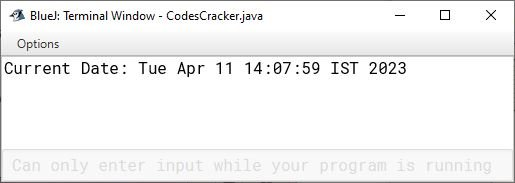This Java program imports the "Date" class from the "java.util" package and defines a class named "JavaDateExample." Within the "main" method, a new "Date" object is created and assigned to the "currentDate" variable using the constructor of the "Date" class. You will learn about constructors later in this Java tutorial series, after covering the basics of object-oriented programming, which are classes and objects.

Now coming to the point, this object represents the current date and time based on the system clock of the computer running the program. Then, the program prints the string "Current Date: " followed by the value of the "currentDate" object using the "System.out.println" method, which outputs the string to the output console.

Another way to print the current date (excluding time) and in the format "YYYY-MM-DD", you can use the "java.time.LocalDate" as shown in the example given below:

```import java.time.LocalDate;

public class DateExample {
public static void main(String[] args) {
LocalDate currentDate = LocalDate.now();
System.out.println("Current Date: " + currentDate);
}
}```

Now the output should be: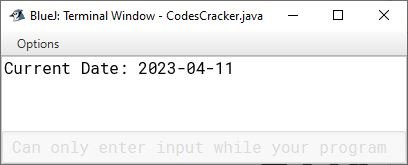However, you can format the date using the "SimpleDateFormat" class based on your requirements. For example:

```import java.text.SimpleDateFormat;
import java.util.Date;

public class DateFormattingExample {
public static void main(String[] args) {
Date currentDate = new Date();

SimpleDateFormat formatter = new SimpleDateFormat("dd-MM-yyyy HH:mm:ss");

String formattedDate = formatter.format(currentDate);
System.out.println("Date: " + formattedDate);
}
}```

In this example, the SimpleDateFormat object is created with a format pattern of "dd-MM-yyyy HH:mm:ss". This format pattern represents the day (dd), month (MM), year (yyyy), hour (HH), minute (mm), and second (ss) of the Date object. So I get the following output this time: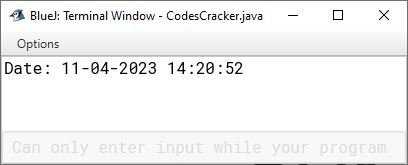## Print the current time in Java

You can format and print the current time in Java with the help of the program above. As an example:

```import java.text.SimpleDateFormat;
import java.util.Date;

public class TimeExample {
public static void main(String[] args) {
Date currentDate = new Date();

SimpleDateFormat formatter = new SimpleDateFormat("HH:mm:ss");

String formattedDate = formatter.format(currentDate);
System.out.println("Current Time: " + formattedDate);
}
}```

The output should be: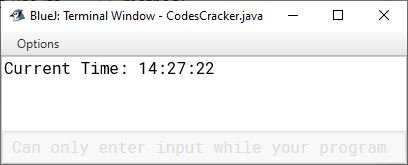Or you can create a separate program using the "LocalTime" class of the "java.time" package. Consider the following Java program as a demonstration of printing the current time in Java.

```import java.time.LocalTime;

public class CurrentTimeExample {
public static void main(String[] args) {
LocalTime currentTime = LocalTime.now();
System.out.println("Current Time: " + currentTime);
}
}```

This program produces the following output: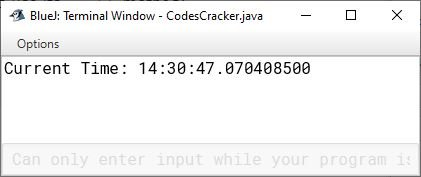I said "this program produces" does not mean that we will get the above output all the time; instead, it depends on the current time when executing this program 😀.

I also created a separate post that contains the programs for printing dates and times in Java.

The following table lists and briefly describes the most commonly used classes when working with date and time in Java.

Class Description
java.util.Date Shorthand for a date and time that can be specified down to the millisecond. In Java 8 and later, the "java.time" API is used instead.
java.util.Calendar Dates and times can be calculated and formatted with this class. However, it often lacks precision and is overly wordy.
java.time.LocalDate Dates without timestamps, such as 2023-04-11, are represented by this class.
java.time.LocalTime Time without a date component, such as 11:45:22.
java.time.LocalDateTime Date and time are represented without regard to timezone.
java.time.ZonedDateTime Represents a date and time, including a time zone component.
java.time.format.DateTimeFormatter Date and time information can be formatted and parsed.

Java Online Test

« Previous Tutorial Next Tutorial »